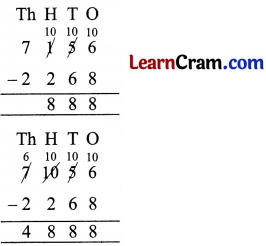# DAV Class 3 Maths Chapter 3 Worksheet 2 Solutions

The DAV Class 3 Maths Book Solutions and DAV Class 3 Maths Chapter 3 Worksheet 2 Solutions of Subtraction offer comprehensive answers to textbook questions.

## DAV Class 3 Maths Ch 3 WS 2 Solutions

Subtract (Borrow wherever necessary):

Question 1.Solution: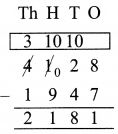Question 2.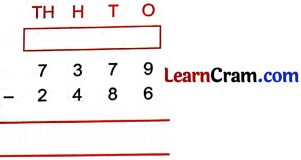Solution: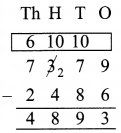Question 3.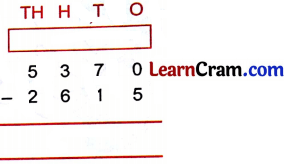Solution:Question 4.Solution:Question 5.Solution:Question 6.Solution: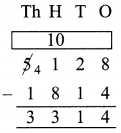Question 7.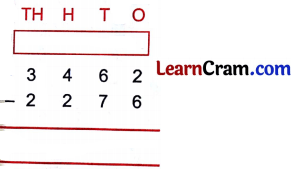Solution:Question 8.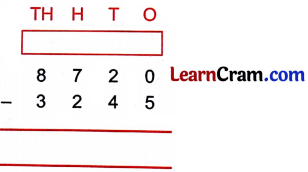Solution:Question 9.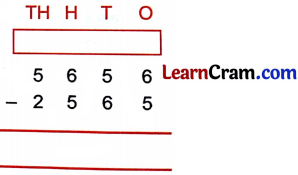Solution:Question 10.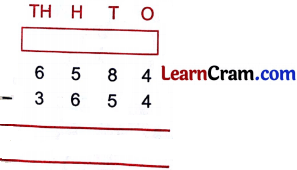Solution: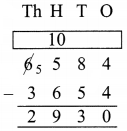Question 11.Solution:Question 12.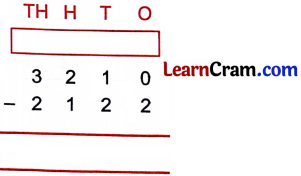Solution:### DAV Class 3 Maths Chapter 3 Worksheet 2 Notes

Subtraction with Regrouping:

Step I:
We cannot subtract 8 from 6 so we borrow 1 ten and change it into 10 tens.

Step 2:
Take another ten from hundred an change it into 10 lens. Then subtract hundreds.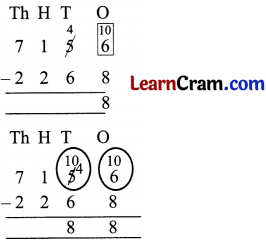Step 3:
We cannot subtract 2 from 0, So we borrow 1 thousand and change it into 10 hundreds.

Step 4:
Now we can subtract thousands.